IF YOU CAN SEE THIS go to /sfa/Nuprl/Shared/Xindentation_hack_doc.html

### Subtyping and Polymorphism

As explained in Equality, a single notation can be used to denote values in multiple types, and a pair of notations can be used to denote distinct values of one type and yet denote a single value in another type.
(One consequence is that one cannot always simply "read off" an intended type from an expression.)

Subtyping: Void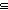A; {x:AP(x) }A; A(x,y:A//E(x,y)); (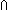x:AB(x))B(a); ATop

Recall that Functions are denoted by Computationally significant expressions that operate on notations for the arguments. It often happens that functional notations working on notations of one type "T" will also "automatically" work on all notations of some other type "S". Or equivalently, the notations of type "S" are also notations of type "T". Then we may say

Def ST ==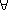x:Sx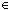T,

and consequently, when ST, then (T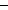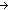A(SA), i.e.f:(TA). fSA.
One obvious way for ST to happen is simply for S to be a subclass of T, e.g.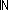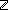, or more generally,
{x:AP(x) }A (see Comprehension Subtypes), and as an extreme case,
VoidA.

But there is also a subtler aspect of subtyping. Recall from Functions that the core criterion for being a notation for a function over a domain type D is that its application to any notations of D denoting the same input value must yield notations for the same output value. Thus,

Thm*f:((mod 2)A). fA

since the notations of "mod k" are just those ofwith certain distinct integer notations becoming identified, namely those x and y such that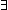m:x-y = m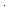k. Thus,(mod k), or more generally,
T(x,y:T//E(x,y)) (see Quotient Types)

Another type constructor intimately related to expressing polymorphism is Intersection,x:AB(x). Here we give six other facts about functions that are polymorphic over all input types. The first says that a function expression completely polymorphic in the domain type may be used as both function and argument in a single application. The second says that if the output type does not depend on the input type, then the function is constant. The third says that identity is the only polymorphic function mapping the domain into itself. The fourth and fifth together say that a function generating a (D List) from a D value, ignoring the choice of D, must generate a list whose length is independent of the input, and all of whose members simply are the input value. The sixth says that the swap function is the only polymorphic C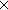DDC function.

Thm*T:Type, f:(D:Type. DT). f(fT
Thm*f:(D:Type. DT), A:Type, a:AB:Type, b:Bf(a) = f(bT
Thm*f:(D:Type. DD), A:Type, a:Af(a) = a
Thm*f:(D:Type. D(D List)), A:Type, a:AB:Type, b:B.
Thm* ||f(a)|| = ||f(b)||Thm*f:(D:Type. D(D List)), A:Type, a,x:Axlist f(a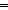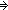x = a
Thm*f:(C,D:Type. CDDC), A,B:Type, a:Ab:Bf(<a,b>) = <b,aBA

See Typing Y for another example.

The type Top

Finally, there is a unit type "Top" whose sole member is denoted by every closed expression. It is called "Top" because Thm* ATop.

NOTE that "Top" is not a type of all values, even though every (closed) expression has type "Top" (thus "whateverTop"); it is just that the semantics is so trivial as to exclude no possible notation (the other extreme of triviality is Void, whose semantics trivially excludes every notation). In particular even though (TopTop) is true, this does not mean that the type Top is a member of Top, since equality in Top has nothing to do with equality between types.

(Rather than building Top in as a primitive it is defined as Def Top == Void(given Void).)

(Nov 2002 - sfa )